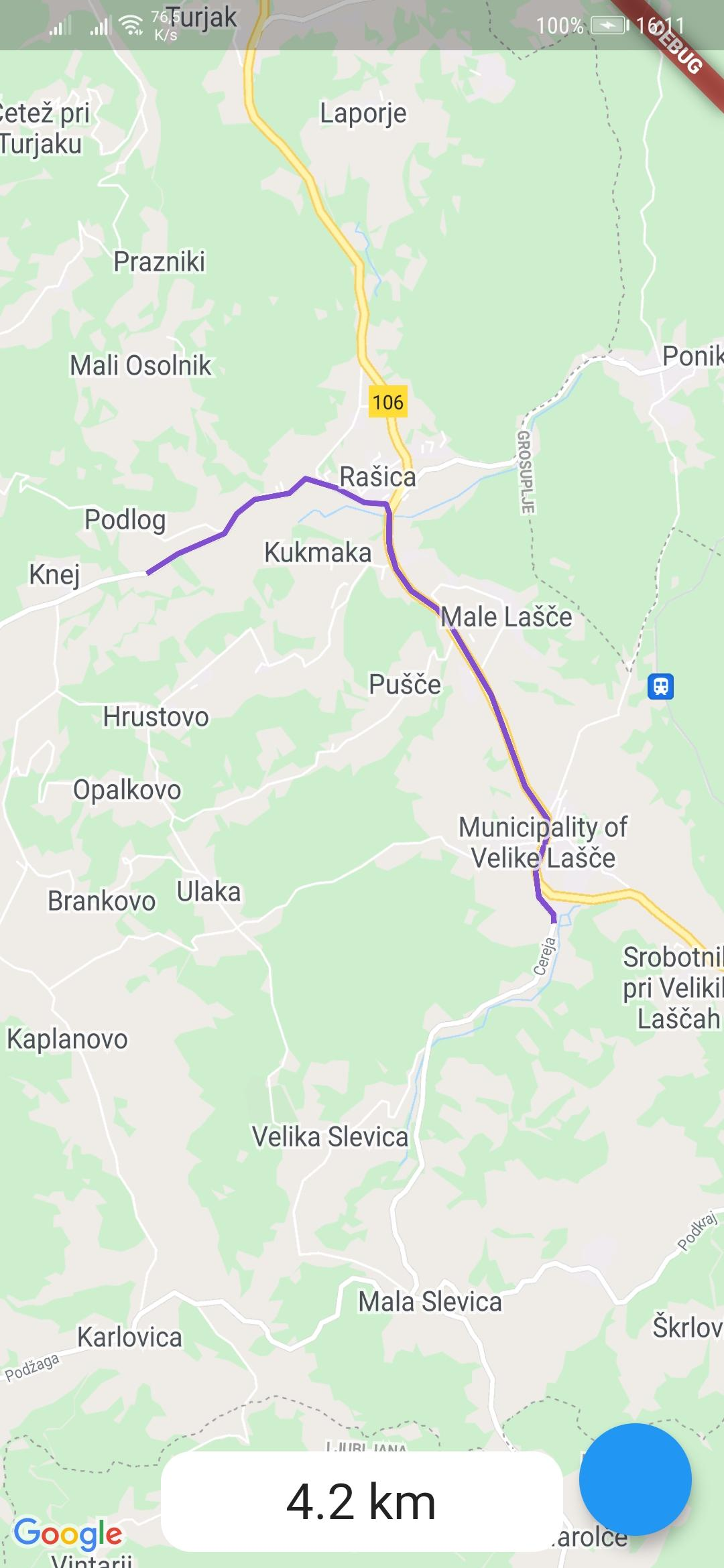This is a package for creating routes on google maps using the directions API from Google. Routes are drawn with help of the flutter_polyline_points package.

## Screenshot## Usage

To use the google_maps_routes package, first depend on it.

Then import it where you want to use it

``````import 'package:google_maps_routes/google_maps_routes.dart';
``````

To use it you need a `List` of points like this:

``````/// LatLng is included in google_maps_flutter
List<LatLng> points = [
LatLng(latitude, longitude),
LatLng(latitude, longitude),
LatLng(latitude, longitude),
LatLng(latitude, longitude),
];
``````

Then you need to instantiate this plugin

``````MapsRoutes route = new MapsRoutes();
``````

To create a route, just call the following function:

``````/// routeName is a string and can be anything; it can include spaces but
/// they will be replaced with a dash
/// example: 'Example route' turns into 'Example-route'

/// color is a Color type and will be used as the polyline color:
/// example: Color.fromRGBO(130, 78, 210, 1.0)

/// googleApyKey is a string and is a google directions API key
/// example: get it at
``````

To use a different travel mode, use the travelMode parameter.

``````/// Options: driving, bicycling, transit, walking
await route.drawRoute(
points,
routeName,
color,
travelMode: TravelModes.walking
);
``````

``````GoogleMap(
polylines: route.routes
)
``````

If you ever want to clear the routes, just call:

``````route.routes.clear();
``````

If you want to calculate the distance of the route use you call the following function with the same `List` of points you used for drawing the route. You can also use it by itself.

``````/// Initialize a calculator
DistanceCalculator distanceCalculator = new DistanceCalculator();

/// Call a function that returns a string
/// Pass it the List of points
/// By default it will return with 1 decimal point but you can pass an
/// option that changes that
distanceCalculator.calculateRouteDistance(points, decimals: 1);
``````